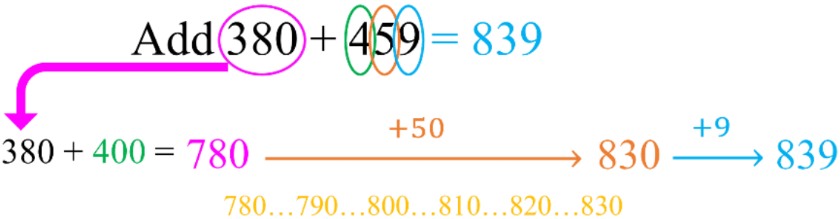#### All in One Place

Everything you need for JC, LC, and college level maths and science classes.#### Learn with Ease

We’ve mastered the national curriculum so that you can revise with confidence.#### Instant Help

24/7 access to the best tips, walkthroughs, and practice exercises available.

0/5
##### Intros
###### Lessons
1. Introduction to Addition Strategies:
2. Mental math and sums of 10
3. Addition strategy #1: friendly numbers with adjustment
4. Addition strategy #2: creating friendly numbers using addends
5. Addition strategy #3: adding on from left to right
6. Addition strategy #4: adding by counting on
0/21
##### Examples
###### Lessons
1. Adding by grouping sums of 10
Add by pairing up sums of 10. Circle the pair and fill in the blanks.
1. 8 + 5 + 2 = ____
10 + ____ = ____
2. 1 + 7 + 3 = ____
10 + ____ = ____
3. 6 + 9 + 4 = ____
10 + ____ = ____
2. Adding by 9 strategy
Add 9 by: adding 10 first, then taking 1 away. Fill in the blanks.
1. 7 + 9 = ____
7 + 10 = ____ - 1 = ____
2. 14 + 9 = ____
14 + 10 = ____ - 1 = ____
3. 38 + 9 = ____
38 + 10 = ____ - 1 = ____
3. Adding by 8 strategy
Add 8 by: adding 10 first, then taking 2 away. Fill in the blanks.
1. 7 + 8 = ____
7 + 10 = ____ - 2 = ____
2. 16 + 8 = ____
16 + 10 = ____ - 2 = ____
3. 54 + 8 = ____
54 + 10 = ____ - 2 = ____
4. Adding using friendly numbers with adjustment
Add by changing one number into a "friendly" number and adjust. Fill in the blanks.
1.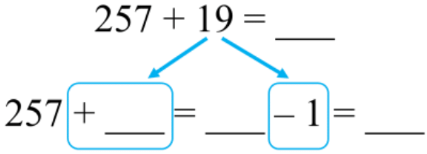2.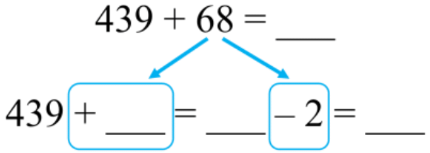3.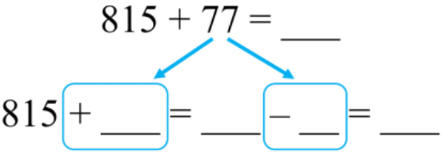5. Adding by making friendly numbers with given addends
Add: make "friendly" numbers using given addends. Fill in the blank.
1.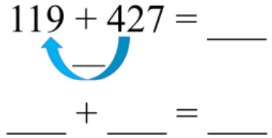2.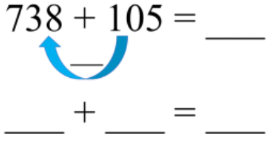3.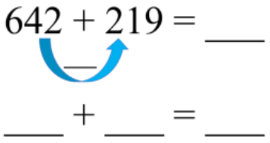6. Adding on strategy
Use the method of adding on from left to right.
1. 553 + 385 = ____
2. 730 + 291 = _____
3. 664 + 148 = ____
7. Counting on from the first number
Add by counting on from the first number
1. 104 + 693 = ____
2. 827 + 335 = _____
3. 568 + 429 = ____
0%
##### Practice
###### Free to Join!
StudyPug is a learning help platform covering math and science from grade 4 all the way to second year university. Our video tutorials, unlimited practice problems, and step-by-step explanations provide you or your child with all the help you need to master concepts. On top of that, it's fun - with achievements, customizable avatars, and awards to keep you motivated.
• #### Easily See Your ProgressWe track the progress you've made on a topic so you know what you've done. From the course view you can easily see what topics have what and the progress you've made on them. Fill the rings to completely master that section or mouse over the icon to see more details.
• #### Make Use of Our Learning Aids###### Practice Accuracy

Get quick access to the topic you're currently learning.

See how well your practice sessions are going over time.

Stay on track with our daily recommendations.

• #### Earn Achievements as You LearnMake the most of your time as you use StudyPug to help you achieve your goals. Earn fun little badges the more you watch, practice, and use our service.
• #### Create and Customize Your AvatarPlay with our fun little avatar builder to create and customize your own avatar on StudyPug. Choose your face, eye colour, hair colour and style, and background. Unlock more options the more you use StudyPug.
###### Topic Notes

In this lesson, we will learn:

• The addition strategies of: (1) using friendly numbers with adjustment, (2) creating friendly numbers using addends, (3) adding on from left to right, and (4) adding by counting on
• Understanding adding by 9

Notes:

• Method 1: change into a "friendly" number and then adjust (by subtracting)
• Round one of the addends to the nearest multiple of 10
• Then adjust by taking away how much you rounded up by to get the answer• Method 2: make a "friendly" number using the addends
• Use the numbers that were given for the addition equation
• Move values from one number to the other number to make a multiple of 10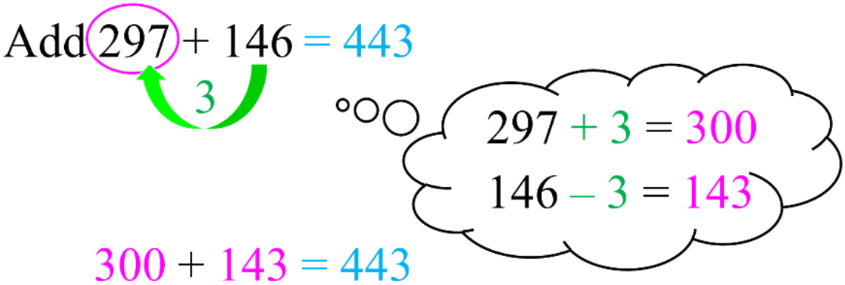• Method 3: adding from left to right
• Add by going through one place value at a time
• i.e. hundreds of 1st # + hundreds 2nd # + tens of 1st # + tens of 2nd # + . . .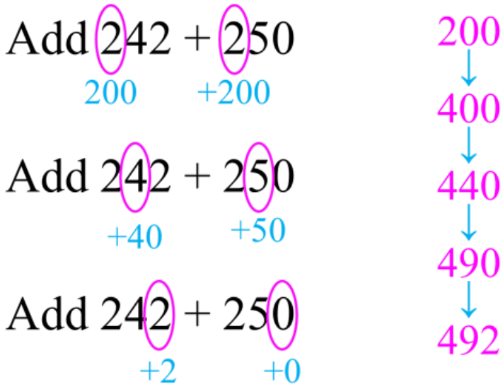• Method 4: adding by counting on
• Start with the first number, then add the second number one place value at a time